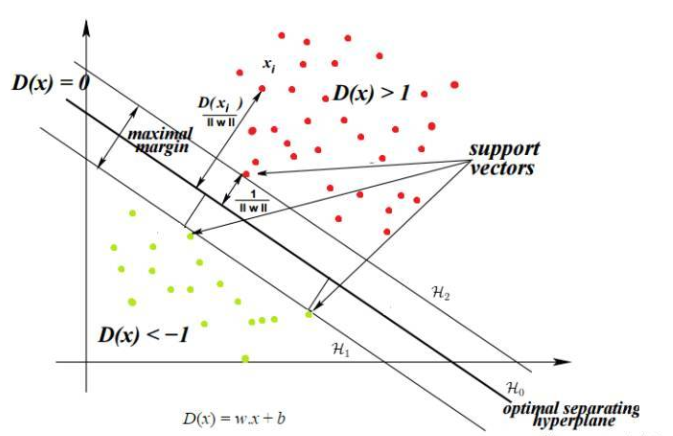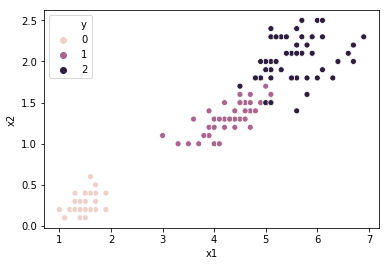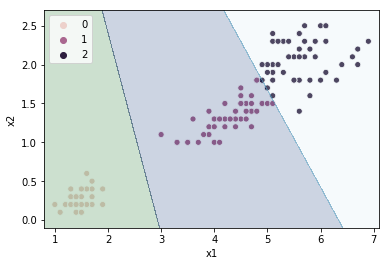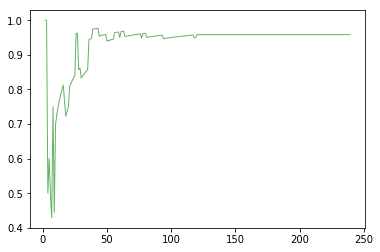# 机器学习实战-支持向量机原理、Python实现和可视化（分类）

## 2.SVM的原理

### 什么是分离超平面？

• 1维数据集，单点表示超平面。
• 2维数据集，线是超平面。
• 3维数据集，平面是超平面。
• 在更高的维度上，就被称为超平面。

SVM的目标是找到最佳分离超平面。那么什么时候分离超平面是最优的？让我们通过一组图来理解最佳超平面。有多个超平面，但其中哪一个是分离超平面？ 可以很容易地看出，线B是比较好地分离这两个类的线，但我们如何计算出来最佳的超平面呢？SVM的目标是找到最佳超平面，因为它不仅分类现有数据集，而且有助于预测未知数据的类。最优超平面是边距最大的平面。

## 3、支持向量机的优缺点

### SVM的一些优点如下：

• 凸优化方法的本质是保证最优性。该解决方案保证是全局最小值，而不是局部最小值
• SVM是一种适用于线性和非线性可分离数据（使用核函数技巧）的算法。唯一要做的是找出正则化项C
• SVM在低维和高维数据空间上工作良好。它能有效地对高维数据集工作，因为SVM中的训练数据集的复杂度通常由支持向量的数量而不是维度来表征。即使删除所有其他训练示例并重复训练，我们将获得相同的最佳分离超平面。
• SVM可以在较小的训练数据集上工作，因为它们不依赖于整个数据。

### SVM的缺点如下：

• 它们不适合较大的数据集，因为在较大的数据集上使用SVM的训练时间可能很高，并且计算量更大。
• 它们在具有重叠类的嘈杂数据集上效率较低。

## 4、Python实现

### 4.1 加载需要用到的模块

import numpy as np
from sklearn import datasets
from sklearn.pipeline import Pipeline
from sklearn.svm import SVC
import seaborn as sns
import pandas as pd
from sklearn.model_selection import cross_val_score
from sklearn.grid_search import GridSearchCV
from sklearn.model_selection import train_test_split
from sklearn.metrics import accuracy_score
import matplotlib.pyplot as plt

### 4.2 初始化数据

iris = datasets.load_iris()
X = iris['data'][:,[2,3]]
y = iris['target']
X = pd.DataFrame(X)
y = pd.DataFrame(y)
data = pd.merge(X,y,left_index=True,right_index=True,how='outer')
data.columns=['x1','x2','y']
h = 0.002
x_min, x_max = data.x1.min() - 0.2, data.x1.max() + 0.2
y_min, y_max = data.x2.min() - 0.2, data.x2.max() + 0.2
xx, yy = np.meshgrid(np.arange(x_min, x_max, h),
np.arange(y_min, y_max, h))
sns.scatterplot(data.x1, y=data.x2,hue=data.y)
X = data[['x1','x2']]
y = data.y## 4.3 线性SVM模型

X_train,X_val,y_train,y_val = train_test_split(X,y,test_size=0.2) #80%和20%划分X和y
clf = SVC(C=0.1,kernel='linear')
clf.fit(X_train,y_train)
y_pre = clf.predict(X)
Z = clf.predict(np.c_[xx.ravel(), yy.ravel()])
Z = Z.reshape(xx.shape)
sns.scatterplot(data.x1, y=data.x2,hue=y_pre)
plt.contourf(xx, yy, Z, cmap=plt.cm.ocean, alpha=0.2)
accuracy_score(data.y,y_pre)accuracy_score(data.y,y_pre)


## 4.4 寻找最好的模型

params = {'C':np.arange(0.1,1,0.1),'kernel':['linear', 'poly', 'rbf', 'sigmoid']}
gsearch = GridSearchCV(estimator = SVC(kernel='linear'),
param_grid = params, scoring='accuracy',iid=False, cv=5)
gsearch.fit(X,data.y)
gsearch.grid_scores_, gsearch.best_params_, gsearch.best_score_params = {'C':np.arange(0.1,1,0.1),'degree':np.arange(1,5,1)}
gsearch = GridSearchCV(estimator = SVC(kernel='poly'),
param_grid = params, scoring='accuracy',iid=False, cv=5)
gsearch.fit(X,data.y)
gsearch.grid_scores_, gsearch.best_params_, gsearch.best_score_

{‘C’: 0.10000000000000001, ‘degree’: 2},

0.9666666666666668)

### 4.5 最优模型

clf = SVC(C=0.2,kernel='rbf')
clf.fit(X,y)
y_pre = clf.predict(X)
Z = clf.predict(np.c_[xx.ravel(), yy.ravel()])
Z = Z.reshape(xx.shape)

ax = sns.scatterplot(data.x1, y=data.x2,hue=y_pre)
ax.legend(loc="lower right")
plt.contourf(xx, yy, Z, cmap=plt.cm.ocean, alpha=0.2)accuracy_score(data.y,y_pre)

### 4.6 学习曲线

scores = []
for m in range(2,X_train.size):#循环2-79
clf.fit(X_train[:m],y_train[:m])
y_train_predict = clf.predict(X_train[:m])
y_val_predict = clf.predict(X_val)
scores.append(accuracy_score(y_train_predict,y_train[:m]))
plt.plot(range(2,X_train.size),scores,c='green', alpha=0.6)#### 请关注我的微信公众号